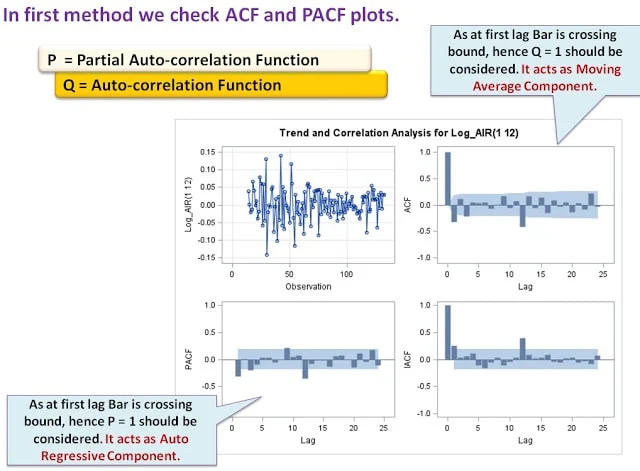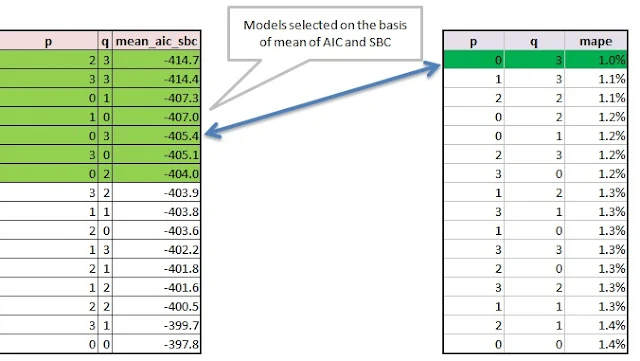# SAS: Time Series Forecasting - ARIMA [Part 3]

In the previous two lessons, we covered how to check the volatility and stationarity in the time series, and how to make the series non-volatile and stationary. We also divided the dataset into two parts: training and validation. Now, we are ready to perform ARIMA modeling on the training dataset. I hope you have gone through and enjoyed learning the previous two articles in the series; if not, then please do so.

Next Step : Model Identification
The order of an ARIMA (autoregressive integrated moving-average) model is usually denoted by the notation ARIMA(p,d,q ) or it can be read as AR(p) , I(d), MA(q)
1. p = Order of Autoregression (Individual values of time series can be described by linear models based on preceding observations. For instance: x(t) = 3 x(t-1) - 4 x(t-2))
2. d = Order of differencing (No. of times data to be differenced to become stationary)
3. q = Order of Moving Average (Number of lagged forecast errors in the prediction equation. Past estimation or forecasting errors are taken into account when estimating the next time series value. The difference between the estimation x(t) and the actually observed value x(t) is denoted ε(t). For instance: x(t) = 3 ε(t-1) - 4 ε(t-2).)
Many of the simple time series models are special cases of ARIMA Model
1. Simple Exponential Smoothing ARIMA(0,1,1)
2. Holt's Exponential Smoothing ARIMA(0,2,2)
3. White noise ARIMA(0,0,0)
4. Random walk ARIMA(0,1,0) with no constant
5. Random walk with drift ARIMA(0,1,0) with a constant
6. Autoregression ARIMA(p,0,0)
7. Moving average ARIMA(0,0,q)

We can do the model identification in two ways :

1. Using ACF and PACF Functions
2. Using Minimum Information Criteria Matrix (Recommended)
Autocorrelation Function (ACF)

Autocorrelation is a correlation coefficient. However, instead of correlation between two different variables, the correlation is between two values of the same variable at times Xt and Xt-h. Correlation between two or more lags.

Partial Autocorrelation Function (PACF)

For a time series, the partial autocorrelation between xt and xt-h is defined as the conditional correlation between xt and xt-h, conditional on xt-h+1, ... , xt-1, the set of observations that come between the time points t and t−h.ARIMA - ACF PACF

ARIMA Procedure
identify var=VariableY(PeriodsOfDifferencing);
estimate p=OrderOfAutoregression q=OrderOfMovingAverage;
where VariableY is modeled as ARIMA(p,d,q) with p = OrderOfAutoregression, d = the order of differencing (determined from PeriodsOfDifferencing), and q = OrderOfMovingAverage.

Using these identified p and q values, we run ARIMA model.
PROC ARIMA DATA= Training ;
IDENTIFY VAR = Log_Air(1,12) ;
ESTIMATE P =1 Q =1 OUTSTAT= stats ;
Forecast lead=12 interval = month id = date
out = result;
RUN;
Quit;

We strongly suggest to follow Minimum Information Criteria Matrix approach though.
Minimum Information Criteria Matrix approach

A MINIC table is then constructed using BIC(m,j) where m=pmin,.......pmax and j=qmin....qmax.ARIMA Orders
We run following code first to get MINIC:
PROC ARIMA DATA= Training;
IDENTIFY VAR = Log_Air(1,12) MINIC;
RUN;Quit;
It would give you the matrix given below. Find the minimum value (largest negative) point in the matrix.ARIMA : MIC

Now we consider the maximum of P(3) and Q(0) suggested by MINIC which is max(3,0) = 3 in this case. And then we iterate ARIMA model for P = 0 to 3 to Q = 0 to 3 (Except 0,0).

```%Macro top_models;

%do p = 0  %to 3 ;
%do q = 0 % to 3 ;

PROC ARIMA DATA= test ;
IDENTIFY VAR = Log_Air(1,12)  ;
ESTIMATE P = &p. Q =&q.  OUTSTAT= stats_&p._&q. ;
Forecast lead=12 interval = month id = date
out = result_&p._&q.;
RUN;
Quit;

data stats_&p._&q.;
set   stats_&p._&q.;
p = &p.;
q = &q.;
Run;

data result_&p._&q.;
set   result_&p._&q.;
p = &p.;
q = &q.;
Run;

%end;
%end;

Data final_stats ;
set %do p = 0  %to 3 ;
%do q = 0 % to 3 ;
stats_&p._&q.
%end;
%end;;
Run;
Data final_results ;
set %do p = 0  %to 3 ;
%do q = 0 % to 3 ;
result_&p._&q.
%end;
%end;;
Run;

%Mend;
%top_models

/* Then to calculate the mean of AIC and SBC*/
proc sql;
create table final_stats_1  as select p,q, sum(_VALUE_)/2 as mean_aic_sbc from final_stats
where _STAT_ in ('AIC','SBC')
group by p,q
order by mean_aic_sbc;
quit;
```
Save AIC and SBC values of all the iterations and choose top 5-7 models with minimum mean(AIC,SBC) values.

Now for all these selected models selected using AIC and SBC average, we calculate MAPE on validation data. We run the ARIMA on validation data with all selected P and Q.
Mean Squared Percentage Error (MAPE) for each model :
MAPE = Abs(Actual – Predicted) / Actual *100

Use the following code to calculate MAPE :

```Proc SQL;
create table final_results_1 as select a.p, a.q, a.date,a.forecast, b.log_air
from final_results as a join validation as b
on a.date = b.date;
quit;

Data Mape;
set final_results_1 ;
Ind_Mape = abs(log_air - forecast)/ log_air;
Run;

Proc Sql;
create table mape as select p, q, mean(ind_mape) as mape from mape
group by p, q
order by mape ;
quit;
```
Results:ARIMA : MAPE
Model with least MAPE is finally your climax model which is p= 0, q=3;
Related Posts
Share
8 Responses to "SAS: Time Series Forecasting - ARIMA [Part 3]"
1.Hi,

Thank your for the detailed explanation of the Time Series Forecasting model, it's really helpful.

Could you please also elaborate, after selecting the model with the least MAPE, how would we predict the value for the next time period i.e. Jan 61.

2.in stead of using Forecast lead=12, you can use a higher number in lead option to forecast further.

3.Hi,

Thank you that u r so elaborately explaining ARIMA.

I have a doubt after reading this article.How can we divide the data set into validation and training data while doing so we lose sequence of time series.

1.You do not lose the sequence of the data. First 70-80% data-set works as a training set and the rest for validation.

4.very nice article....

5.Hi...
Really nice. so elaborately explained ARIMA.appreciate your work..

I have a small doubt,if i change my testing or validation data my MAPE will get changed ,So is there any way to make sure that my final model is consistent ..???

and also if you have any knowledge on ARIMAX (x-any additional variable,say macroeconomic variable ) and if you can share any example on that i would really appreciate that..

6.Hi, a typo here that the MAPE should be mean ABSOLUTE percentage error, not SQUAREd.

7.When I run this on my data, the model with the lowest MINIC value was not the model with the lowest MAPE value. In this situation which would you pick? Ideally should the same model have the lowest MINIC and MAPE value?

Next → ← Prev
Looks like you are using an ad blocker!

To continue reading you need to turnoff adblocker and refresh the page. We rely on advertising to help fund our site. Please whitelist us if you enjoy our content.